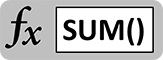# SUM Fundamentals – Make It All Add UpThe SUM function is a simple but important one. It forms the basis for a number of powerful and sophisticated tools including SUMIF and SUMIFS that we will explore later. You have to walk before you can run, though, so let’s take a look at the fundamentals of the SUM function…

Addition is the most common activity you’ll do in Excel, tallying rows, columns, and cells into sub-totals and totals. It’s built into the core of the program – you can do it without using any functions.

`=23+45`

Adds 45 to 23 and calculates to 68.

`=A1+12`

Adds 12 to the contents of cell A1.

`=A1-B1 `

Subtracts the contents of B1 from A1.

## SUM Function Basics

Direct operations work great when you want to make short work of simple calculations. When things get more complicated, you’ll want to use the SUM function. The syntax for SUM is as follows:

`=SUM(number1,[number2],…)`

This means that function needs at least one number or cell reference to compute. After that, you can continue to specify additional numbers and references for the function to add together. This seems simple enough! The same calculations we did above with direct operations can be done with the SUM function.

`=SUM(23,45)`

Adds 45 to 23 and calculates to 68.

`=SUM(A1,12)`

Adds 12 to the contents of cell A1.

`=SUM(A1,-B1)`

Subtracts the contents of B1 from A1.

Look closely at that last example – it reveals a secret about how the SUM function works that makes it much more powerful. SUM means addition, but we were able to subtract B1 by putting a minus sign in front of it. The SUM function did a calculation inside the input! This means you can do all kinds of pre-calculation right in the function. This can save lots of time and space on your worksheet, especially if you only need a calculation once or twice. For example, you can:

Change sign by putting a “-” (minus) sign in front of a number or cell reference:

`=SUM(-A1,-B1)`

Multiply or divide with “*” (asterisk) and “/” (forward slash):

`=SUM(A1*B1,C1/D1)`

Combine operations with parentheses:

`=SUM((A1-B1)/(C1+4),D1)`

## Do More With SUM Ranges

This is okay, but so far we have only duplicated what can be done with direct operations. The best thing about the SUM function is that you can input adjacent groups of cells to be added together in one step. Excel calls these ranges. For example, you can:

Add cells A1, B1, and C1:

`=SUM(A1:C1)`

Add cells A1 through A25:

`=SUM(A1:A25)`

Add cells A1, B1, A2, and B2:

`=SUM(A1:B2)`

You can even add entire columns:

`=SUM(A:A)`

And rows:

`=SUM(12:12)`

Summing ranges is powerful, because if you add cells in the middle of the range, the SUM function automatically re-calculates to add it to the group. When you are building large worksheets that will grow and change over time, using SUM with ranges will save you enormous amounts of time!

## Embedded Functions in SUM

Finally, you can insert entire functions inside a SUM input. COUNT is a function that tells you how many cells are not blank. It can take ranges as inputs just like SUM. Therefore, you could add the output of a COUNT function inside a SUM function.

`=SUM(COUNT(A:A),B1:B5,6)`

Adds cells B1 through B5 and the number 6 to the number of non-blank cells in column A.

The SUM function is the simplest of a family of functions based around summation. The others include SUMIF, SUMIFS, SUMPRODUCT, and they will form the basis of your toolkit for building amazing models in Excel. Look for more from the SUM function family soon!

## Get the latest Excel tips and tricks by joining the newsletter!Andrew Roberts has been solving business problems with Microsoft Excel for over a decade. Excel Tactics is dedicated to helping you master it.

Join the newsletter to stay on top of the latest articles. Sign up and you'll get a free guide with 10 time-saving keyboard shortcuts!

## One thought on “SUM Fundamentals – Make It All Add Up”

1. Mohan says:

How to do average of below format in ex-cell sheet and drag it for rest
1 st cell in row avg of A1A2B1B2
2 nd cell in row avg of B1B2C1C2
3 rd cell in row avg C1C2D1D2 and drag it for rest